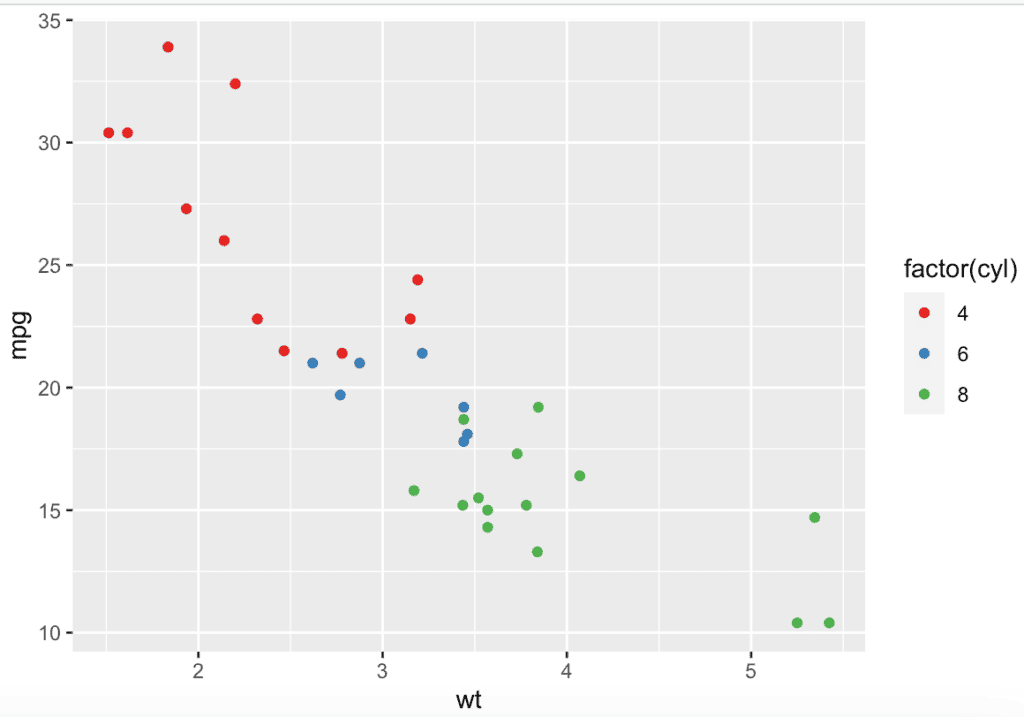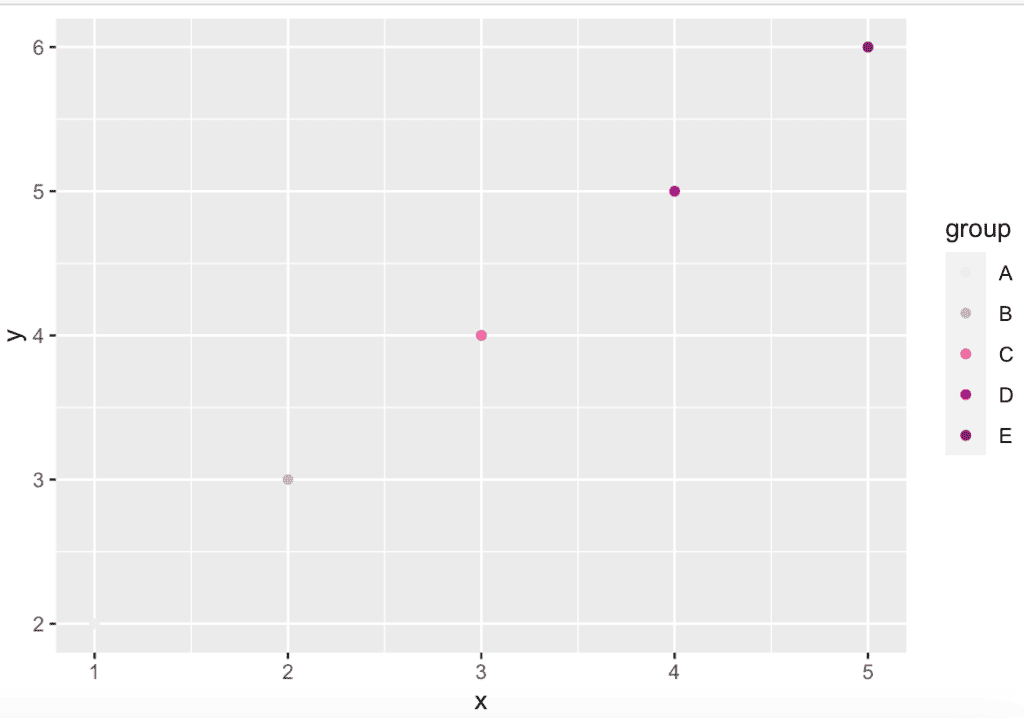# What is scale_colour_brewer() Function in R

The scale_colour_brewer() is an R function from the ggplot2 package that provides sequential, diverging, and qualitative color schemes from ColorBrewer. These are particularly well suited to display discrete values on a map.

### Syntax

``````scale_colour_brewer(
...,
type = "seq",
palette = 1,
direction = 1,
aesthetics = "colour"
)``````

### Parameters

type: It is one of “seq” (sequential), “div” (diverging), or “qual” (qualitative)

palette: If a string will use that named palette. If a number will index into the list of palettes of the appropriate type. The list of available palettes can be found in the Palettes section.

direction: It sets the order of colors in the scale. If 1, the default colors are as output by RColorBrewer::brewer.pal(). If -1, the order of colors is reversed.

aesthetics: The character string or vector of character strings listing the name(s) of the aesthetic(s) that this scale works with.

: Other arguments.

### Example 1

``````library(ggplot2)

ggplot(mtcars, aes(x = wt, y = mpg, colour = factor(cyl))) +
geom_point() +
scale_colour_brewer(palette = "Set1")``````

OutputIn this code example, we created a scatter plot of mpg vs wt from the mtcars dataset. The points are colored based on the number of cylinders (cyl) using the scale_colour_brewer() function with the Set1 palette.

### Example 2

``````library(ggplot2)

# Create a sample data frame
data <- data.frame(x = 1:5, y = 2:6, group = c("A", "B", "C", "D", "E"))

# Create a scatterplot with different colors for each group using the
# RdPu palette
ggplot(data, aes(x, y, color = group)) +
geom_point() +
scale_colour_brewer(type = "qual", palette = "RdPu")``````

OutputIn this example, we created a simple data frame with x and y variables and a categorical grouping variable called “group”.

In the next step, we created a scatterplot with points colored by group using the geom_point() and aes() functions.

Finally, we use scale_colour_brewer() to specify that we want to use a qualitative palette called “RdPu” from the ColorBrewer website.

The type argument is set to “qual” to indicate we want to use a qualitative palette.

That’s it.